# Percentage second harmonic distortion calculation?

Thread moved from the technical forums, so no Homework Help Template is shown
A voltage amplifier ideally should have the input-output relationship of V₀ = 100vi but in practice the relationship is V₀ = Vi (98 + 2Vi).

Calculate the %age second harmonic distortion presents in the amplifiers output for a sinusoidal input of 10mV r.m.s.

Hi everyone! Can anyone please confirm that the following is correct or at least somewhere near?

Voltage Output:

Vo = 98vi + 2vi²
Vo = 98 x 10 + 2 x 10²
Vo = 1180mV

%age Second Harmonic:

%age of Second Harmonic Distortion = Amplitude of Second Harmonic / Amplitude of fundamental x 100

%age of Second Harmonic Distortion = 200/1180 x 100 = 16.95%

BvU
Homework Helper
Hello Callum,Can''t oblige, for two reasons: 1 PF is not for stamp-approving solutions, and b) you didn't calculate the amplitude of the second harmonic.

sorry about that ill keep it in mind for the future. Thanks for point b), I knew I must of misunderstood something somewhere.

jim hardy
Gold Member
Dearly Missed
Sounds like a freshman trig exercise.

If input = a 10 millivolt rms sinewave
then
it's Vi = 0.01414sin(wt) volts..

You didn't square that.

Here's a picture.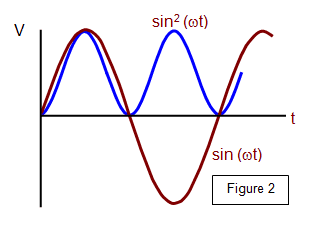Take a look back at your trig identities involving sin2 .

#### Attachments

•Petersilien and dlgoff
Sounds like a freshman trig exercise.

If input = a 10 millivolt rms sinewave
then
it's Vi = 0.01414sin(wt) volts..

You didn't square that.

Here's a picture.

View attachment 226534

Take a look back at your trig identities involving sin2 .
The blue curve in Figure 2 seems to be sin (2wt) - not sin^2(wt)

jim hardy
Gold Member
Dearly Missed
The blue curve in Figure 2 seems to be sin (2wt) - not sin^2(wt)
That's what happens when you square the sin function. Transcendental functions are just that way, different from algebraic ones.

Transcendental function

A transcendental function is an analytic function that does not satisfy a polynomial equation, in contrast to an algebraic function. In other words, a transcendental function "transcends" algebra in that it cannot be expressed in terms of a finite sequence of the algebraic operations of addition, multiplication, and root extraction.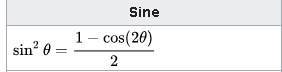Does blue curve reflect half amplitude, double frequency, and constant offset ?
What does right side of that evaluate to when θ = 0 ? π/4 ? π/2 ?

As i said, go back to your trig identities.

old jim

#### Attachments

•Petersilien and Bystander
That's what happens when you square the sin function. Transcendental functions are just that way, different from algebraic ones.

Does blue curve reflect half amplitude, double frequency, and constant offset ?
What does right side of that evaluate to when θ = 0 ? π/4 ? π/2 ?

As i said, go back to your trig identities.

old jim
Sounds like a freshman trig exercise.

If input = a 10 millivolt rms sinewave
then
it's Vi = 0.01414sin(wt) volts..

You didn't square that.

Here's a picture.

View attachment 226534

Take a look back at your trig identities involving sin2 .
Thanks Jim! much appreciated, I'm just getting back into higher education after a few years out and somethings take me awhile to get my head round.

•Petersilien and jim hardy
jim hardy
Gold Member
Dearly Missed
I'm just getting back into higher education after a few years out

Bravo for you !
Transcendantals "Transcend" everyday arithmetic. The original math pioneers calculated them using series, as did the very early electronic calculators via rate multipliers.

•Callum Plunkett and Petersilien
Of course, it works that way.

But, still something does not work, not in the trig side, but in the THD calculation:
maybe I missed something, but I'm not sure that in a second harmonic residual there is a DC component, as formulas above would suggest.

Besides, my interest is about how to calculate second harmonic distortion,
starting instead from a sinusoidal signal, where, i.e.
the amplitude of the upper side is 100,
and the amplitude of the lower side is 90.

Would you have any suggestion on formulas to use?

Thank you for the patience

The Electrician
Gold Member
Of course, it works that way.

But, still something does not work, not in the trig side, but in the THD calculation:
maybe I missed something, but I'm not sure that in a second harmonic residual there is a DC component, as formulas above would suggest.

Besides, my interest is about how to calculate second harmonic distortion,
starting instead from a sinusoidal signal, where, i.e.
the amplitude of the upper side is 100,
and the amplitude of the lower side is 90.

Would you have any suggestion on formulas to use?

Thank you for the patience
What do you mean by "upper side" and "lower side"? Are you referring to the numerator and denominator of a fraction? If so, you should use those words rather than "upper side" and "lower side". Or, if you're referring to upper and lower sidebands, use those words.

•Petersilien
jim hardy
Gold Member
Dearly Missed
I'm not sure that in a second harmonic residual there is a DC component, as formulas above would suggest.

Math says it's there but we don't often encounter it in practice because in most circuits it decays away pretty quickly. So we tend to disbelieve the math and that's always a mistake.

https://www.intmath.com/fourier-series/5-harmonic-analysis.php

and http://msp.ucsd.edu/techniques/v0.11/book-html/node185.html

One place 2nd harmonic's DC content is problematic is transformer inrush when the switch gets closed at a zero crossing...
https://cdn.selinc.com/assets/Literature/Publications/Technical Papers/6615_LowSecondHarmonic_BK_20140210_Web.pdf?v=20160224-120704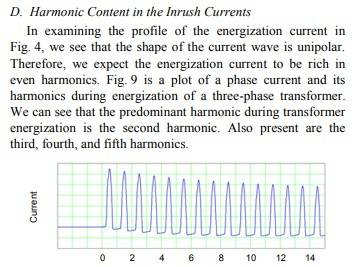The 'Why' behind that transformer inrush:
Flux in an inductor is integral of applied voltage, and when you integrate there's always a constant of integration.
Try evaluating ∫sin(x)dx starting at x=zero. It never goes negative.
https://m.wolframalpha.com/input/?i=integrate+sin+x+dx+from+x=0+to+pi&lk=3
But start at a peak, π/4, and it's zero centered.

old jim

#### Attachments

Last edited:
•Petersilien
jim hardy
Gold Member
Dearly Missed
Would you have any suggestion on formulas to use?
I do not.
Once i found in a 1940's textbook a procedure to find the first few Fourier coefficients from a graphical plot of a function. My brief Google search hasn't turned up anything similarly useful, authors today seem all to go the other way ..

•Petersilien
I apologize - context is missing.

I was looking for a procedure to calculate 2d harmonic distortion, starting from graphic characteristics of a vacuum tube (i.e. a triode), and maybe, from the output waveform, as can be seen on an oscilloscope, and amplitudes of upper and lower half of sine wave measured.

(That’s what I was meaning in the previous post, I wasn’t referring to the terms of the formulas).

In the Ia / Va graphic of a triode, you can easily appreciate the difference of Va (or Ia) along the load line, starting from the operating point, for a given variation of Vg (i.e. +/- 2V).

So you can find, let’s say, a swing of +50V and -40 V along the load line.

This asymmetric distortion will be mainly second harmonic,

I wonder if it’s too simple a rough evaluation of THD as

THD = (50-40)/50 % = 20%

Or, maybe,

THD = (50-40)/((50+40)/2) % = 22%

There is no need of absolute precision, because the purpose is to evaluate the performance of different operating points and load lines, on the same graphic, for a quick comparison.

I hope it’s comprehensible, otherwise I’ll draw a graphic.

jim hardy
Gold Member
Dearly Missed
Just to make a rough estimate i'd reason this way

Even harmonics affect positive and negative peaks oppositely - peaking one and flattening the other if you like.

Your -40 to +50 volt sine-like wave is approximately a +/- 45 volt one with positive peaks increased to 50 and negative ones flattened to 40, suggesting about 5 volts of even harmonic which we can ascribe all to 2nd . for estimating purposes.
45 sin(wt) +5sin(2wt) - (any resulting DC offset) ? 5/45 = 11% ?

Wikipedia: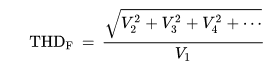with only 2nd harmonic 5/45 works....

what ya think ? Not a bad SWAG ?

#### Attachments

•Petersilien
Well, it makes a lot of sense, your help is precious indeed

It seems we cannot escape the DC component!

But not important, as we are interested in the steady state.

BTW, I found in the fourth edition of RCA Radiotron (1953) 13.1 pag. 549 Section 2 – Class A Single Triode the formula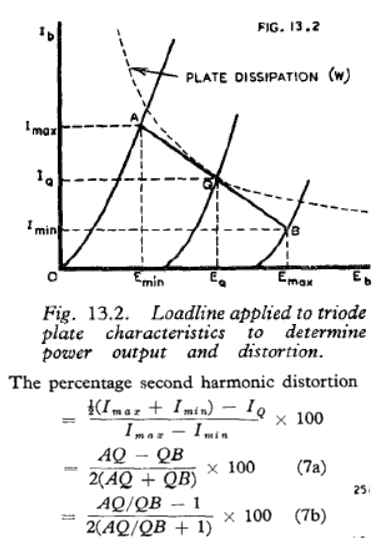but there is no explanation, nor why is taken the anodic current instead of anodic voltage, that we usually observe (and use).

The formula seems the same I used (which I should have divided by 2 – so would equal your result), except for the inversion of division terms,

but your interpretation looks more appropriate.

Thank you indeed!

#### Attachments

jim hardy
Gold Member
Dearly Missed
why is taken the anodic current instead of anodic voltage,
Usually the load is a resistor so current translates linearly to signal voltage. And their ordinate is current,,,

Their logic is probably better than mine. My alleged brain starts with the simplest of basics then refines I didn't linger to refine this, just wanted to get you started thinking in simple steps..

NIce find on the RCA manual. Mine's about a 1962 , if it surfaces i'll see what it says.

Fun subject, thanks !

•Petersilien
Thank to you, I eventually got it stright ! :)

Merlin3189
Homework Helper
Gold Member
A few observations:
If input = a 10 millivolt rms sinewave
then it's Vi = 0.01414sin(wt) volts..
Perfectly true, but unnecessary, since the √2 factor always cancels out. Peak values are just as good.

Petersilien said:
I'm not sure that in a second harmonic residual there is a DC component, as formulas above would suggest.

jim hardy, said: Math says it's there (the DC offset) but we don't often encounter it in practice because in most circuits it decays away pretty quickly. So we tend to disbelieve the math and that's always a mistake.

Merlin says, yes it is there, but it doesn't decay. It is always removed in AC coupled circuits, but so long as fundamental is being fed into the circuit, 2nd harmonic and DC offset are being generated. In a DC coupled circuit I think the DC component produced by non-linearity in one stage would alter the biasing of the following stage. This could manifest itself as a variation in second stage operating point, depending on the AC amplitude of the signal to the first stage.

I think the transformer situation is different, because, as Jim says, by suitable (or unsuitable!) choice of timing you effectively input a DC pulse. While I agree again with Jim on the, " ∫sin(x)dx starting at x=zero. It never goes negative.", the average value tends to zero as the number of cycles increases.

It is also different in that the DC offset is the cause of the 2nd harmonic generation by moving the core to an asymmetric operating point on the BH curve.

As for the original Q, it seems to me that one can simply use Jim's trig relation to see the relative amplitude of the 2nd harmonic is 1/2, weighted by the 2:98 ratio.
If Vi = Asin(f)

## V_o = 98 A sin(f) - 2 A sin^2(f) = 98 A sin(f) - 2 \frac {A} {2} cos(2f) +2 \frac {A} {2} ##
## \ \ \ \ \ = A ( 98 sin(f) + sin(2f -π/2) + 1) ##

So ## THD = \frac {1} {98} ## , the DC being irrelevant for any THD formula I've found. (Which is good, since it's not a harmonic.)

For the derivation from the asymmetric peaks of the composite waveform, I think Jim has it spot on. I think the RCA formula is that, but it's a pity they don't bother anywhere in their 1400 pages to explain that formula.
They also give the formula, ## THD =\frac {√Σ( harmonics^2) } { √(fundamental^2+ Σ(harmonics^2)) } ##
which every other place I've looked now gives as ## THD =\frac { √Σ( harmonics^2) } {fundamental} ##

•Petersilien and jim hardy
The Electrician
Gold Member
I think the RCA formula is that, but it's a pity they don't bother anywhere in their 1400 pages to explain that formula.
They also give the formula, ## THD =\frac {√Σ( harmonics^2) } { √(fundamental^2+ Σ(harmonics^2)) } ##
which every other place I've looked now gives as ## THD =\frac { √Σ( harmonics^2) } {fundamental} ##
Have a look here: https://en.wikipedia.org/wiki/Total_harmonic_distortion

This place gives both versions.

Reference 3 at that location gives a rationale for using THDF in connection with power electronics. In the U.S., THDR has been consistently used in the audio field.

•Merlin3189, Petersilien and jim hardy
Thanks to all for the useful contribution :)

•jim hardy
jim hardy
Gold Member
Dearly Missed
Sorta an aside but
looking for something else i stumbled across two old references to graphical methods of harmonic analysis.
Since FFT became a reality nobody does it anymore.

Actually that one predicts distortion in a transformer from its BH curve, deriving the Fourier coefficients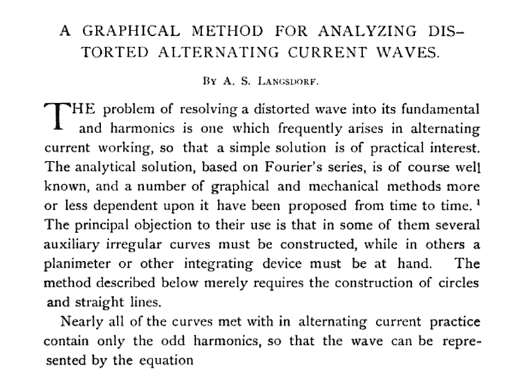"Slow Fourier Transform" ?

old jim

#### Attachments

Thank you for the invaluable reference !•jim hardy